Transcripted Summary

In this chapter we'll be learning about loops.

A loop is a useful construct for when you'd like to repeat the same actions any number of times.

Whether you have a list or a dictionary, with 100 items or a million items, you can use a loop to help you work with that data.

### # For Loops

A `for` loop is useful when you'd like to iterate over each item in a list. The `for` loop allows you to repeat your action for every item in the list or for a specified number of items in the list.

For example, in this list of fruits, we have an apple, an orange, and a pear.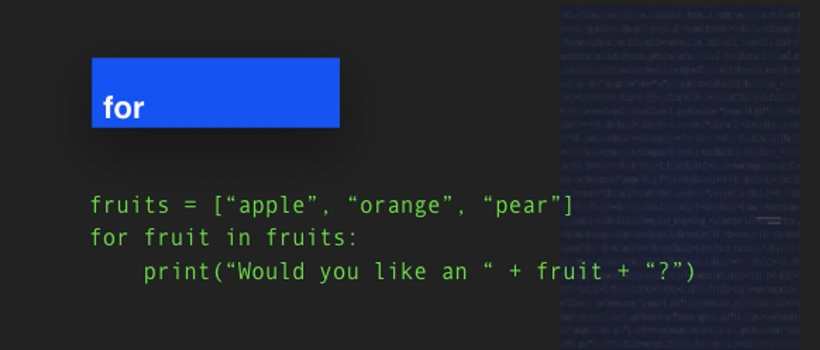For every fruit in that list, we will print a sentence that says:

• Would you like an apple?
• Would you like an orange?
• Would you like a pear?

All 3 of those sentences will be printed with that single line of code.

We'll get started with creating some `for` loops.

We'll get started with this list of fruits, and we're going to make it a pretty short list so that way that we can get to the looping part.

So, here's our list of `fruits`, and we have 5 fruits.

To use our `for` loop, we start with the word `for` and then we can type any variable here. A lot of tutorials that you see will say `for i` in the name of the list of objects.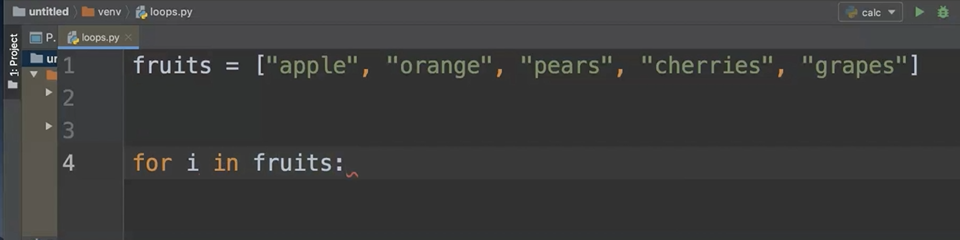I feel like the “i” isn't as clear, so when I'm writing code, if I can make the element clearer, then I do.

And so, in this instance I want it to say `for fruit in fruits`.

Now, why do I choose to say that?

A `for` loop is really something that iterates or repeats itself, loops itself over an object, and this object is a list.

So, for each fruit in the list of fruits, something is going to happen. And that something is whatever we decide to put into our for loop.

To me, for each item is clearer when I name the item. If just using `i` is clearer to you, then you can definitely use that. Both will work.

In this `for` loop, we're going to print a message and we're going to use that single `fruit` variable so that way that each fruit is printed.

``````fruits = ["apple", "orange", "pears", "cherries", "grapes"]

for fruit in fruits:
print("Would you like {} ?".format(fruit))
``````

Let's go ahead, run this code.

It runs so fast that it's actually hard to catch what happened, but you can see it printed out every item.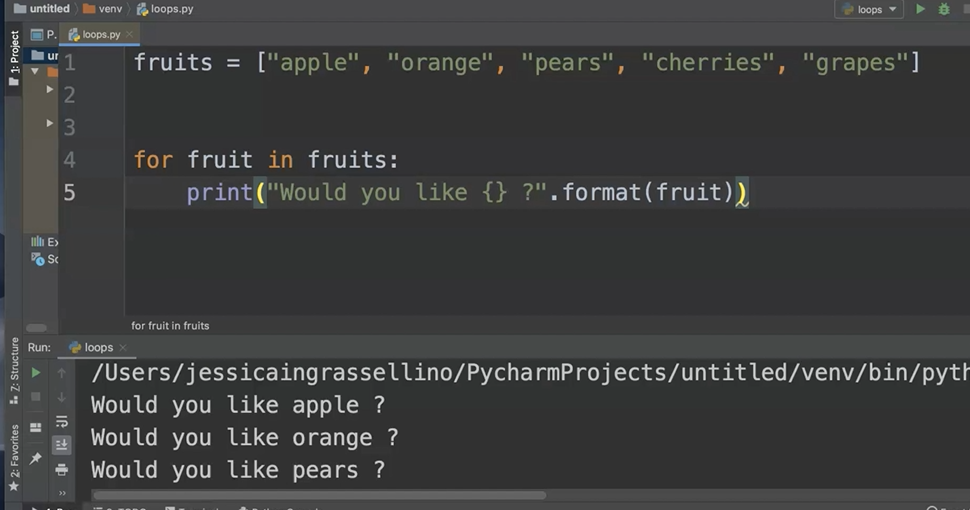It doesn't matter how long the list is, it will print out the items.

For those of you who've seen other tutorials with the `i`, let's switch it up and replace `fruit` with an `i`.

The only thing we need to make sure to do is in the place where we want the individual item, we'll put the “I” instead of “fruit”.

``````for i in fruits:
print("Would you like {} ?".format(i))
``````

I'll go ahead and clear console and then press Run so you can see it's actually going to work in the exact same way.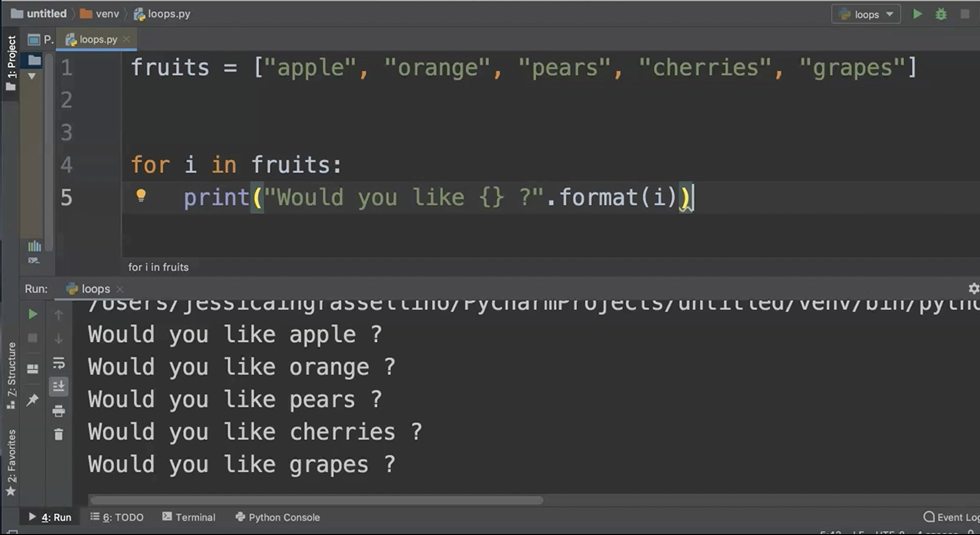Whatever variable is in this position in your `for` loop, we'll pull from whatever individual object is in the group of objects.

Another example of a `for` loop that we can try is looping over a range of numbers.

We'll take a look at the Python `range` function as we do this.

First, we'll start with our `for` statement and then we'll name our individual variable `number`.

What we are asking this loop to do is to go over a range of numbers — `range` is a built-in Python function.

We can assign the range by assigning the first number, a comma, and then assigning the ending number. The ending number is not included.

``````for number in range(1,11):
print("Number {}".format(number))
``````

Our output for this loop will include a String that prints the word “Number”, and then the integer.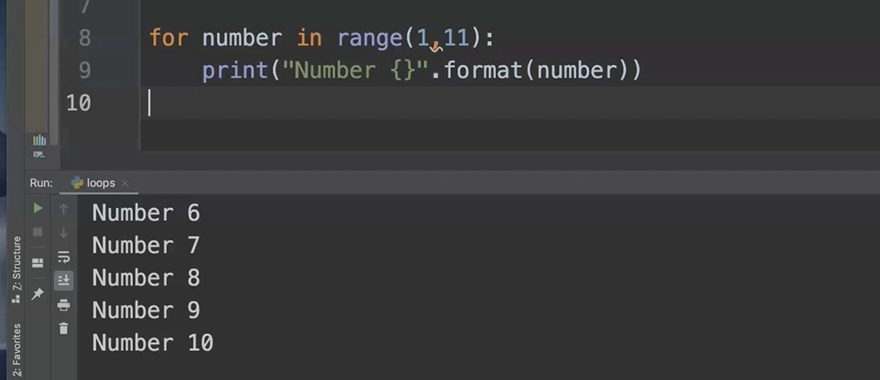You'll notice that we print 1 line for every number from 1 through 10, not including number 11.

### # While Loops

A `while` loop will run any time a condition remains true.

In this example, you'll see that we've set the temperature variable to equal 37.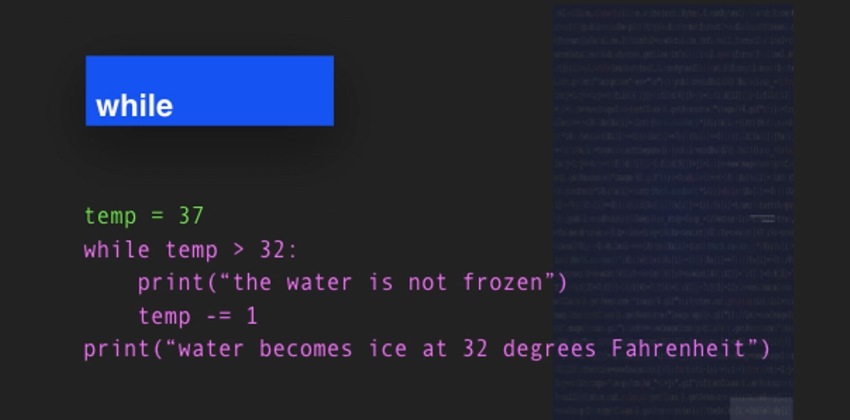While the temperature is greater than 32 the sentence "the water is not frozen" will print and the temperature will be decreased by 1 degree.

When it's over, the sentence "water becomes frozen at 32 degrees Fahrenheit" will print and the loop will stop running.

Let's do a sample of a `while` loop.

In this script that we write, we will set a temperature in Fahrenheit to 40 degrees.

Then we'll make a loop that says, "while the temperature is greater than 32 degrees, we can print a message."

If we run this program now, we will have an infinite loop.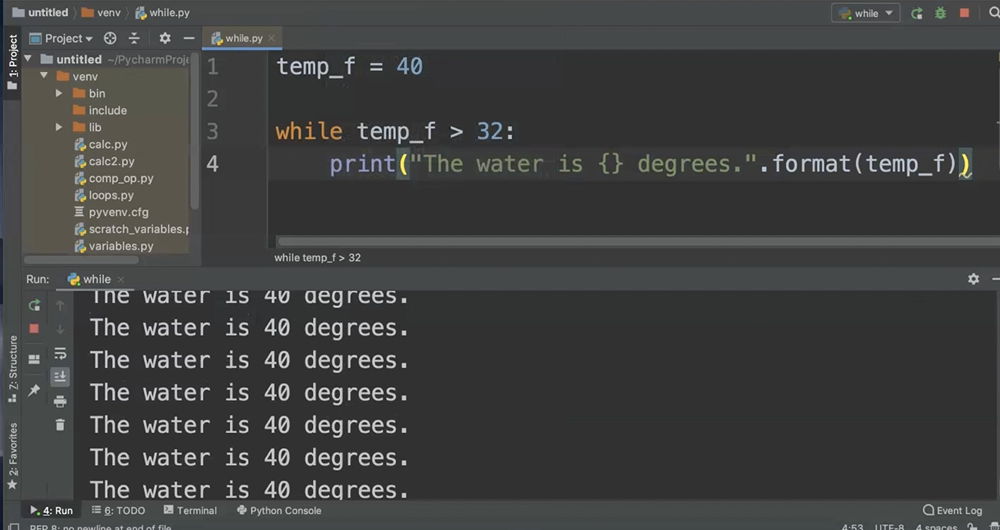An infinite loop is a loop that doesn't have any stopping point. It just keeps going.

So, I'm going to go ahead and stop this loop and clear the console.

I need to create a way for this loop to stop and for the temperature variable to change.

For this sample, I'm going to use the Python decrement operator (`-=`), and all that does is decrease the variable by whatever number I choose.

I'm going to choose to decrease the temperature by 1 for this script.

``````temp_f = 40
while temp_f > 32:
print("The water is {} degrees.".format(temp_f))
temp_f -= 1
``````

And now I'll run it again.

You'll notice that since 33 is greater than 32, it's the last line that prints. And there's a line printed for every number between 40 and 33.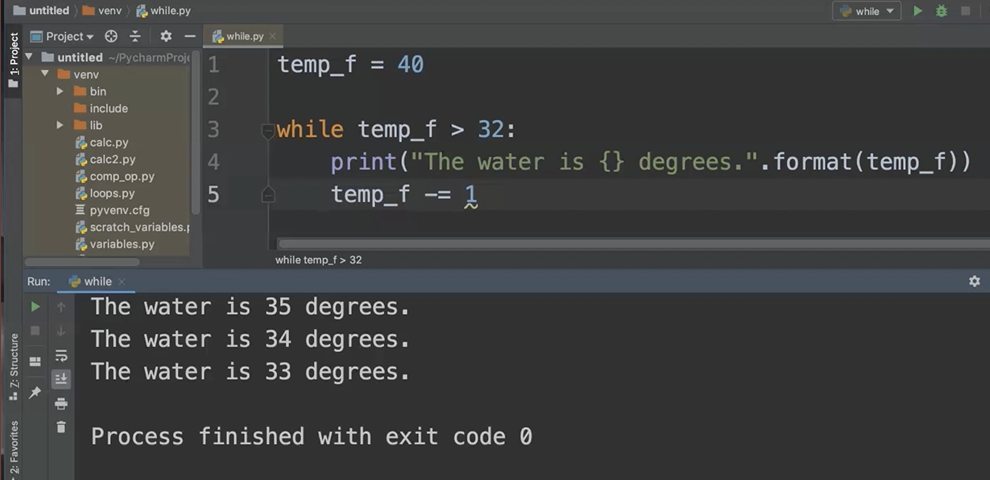So, this is a simple example of how a `while` loop works.

### # Loop Control

You can control the flow of a loop with some loop statements. Python includes `break`, `continue`, and `pass`.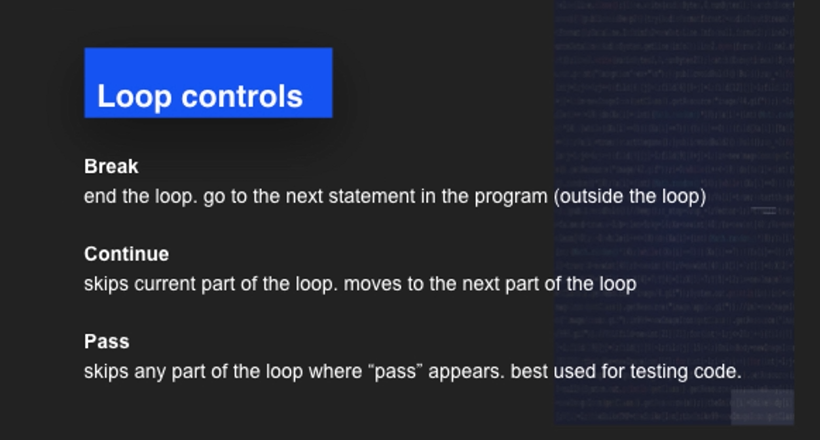• The `break` statement indicates that when you see it, the loop should end and go on to the next statement in the program that is outside of the loop.

• The `continue` statement skips the current part of the loop and moves on to the next part of the loop.

• The `pass` statement skips any part of the loop where `pass` appears. This is best used for testing code, but make sure you don't forget to remove the `pass` statement when you're ready for your code to go into production.

Now we'll take a look at the ways that you can control loops.

We will look at 3 statements: the `break`, the `continue`, and the `pass`.

We'll use the loop code that we used for the `while` loop.

NOTE

If you have that file saved, you can go ahead and open it, and if you don't, go ahead and pause the video and copy the code that's on the screen now (or grab it from the Git Repo listed in the resources).

### # Loop Control: Break

First, we'll try out the `break`.

We can add the `break` by adding an `if` statement.

``````temp_f = 40
while temp_f > 32:
print("The water is {} degrees.".format(temp_f))
temp_f -= 1
if temp_f == 33:
break
``````

What I'm going to do is say if our temperature is equal to 33, then break the loop.

Now I'll go ahead and run the code.

You'll notice that everything before 33 degrees printed out.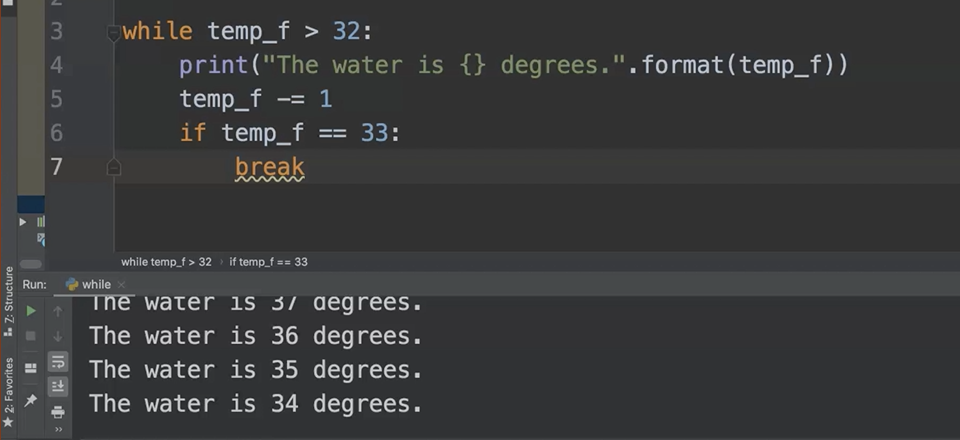And once the temp became equal to 33, the print statement stopped printing and the loop stopped running.

### # Loop Control: Continue

Now we'll work with the `continue` function.

We'll use the `continue` function in a `for` loop. We'll add a `print` statement to help us debug.

``````for number in range(1,11):
if number == 7:
print("We're skipping number 7.")
continue
print("This is the number {}.".format(number))
``````

Indenting

Please make notice of the fact that we've indented all of the code that we want to happen in our `if` statement another 4 spaces. Then the code that we'd like to happen for every number is aligned with the indent of the `if` statement. Indenting is one of those things that can be really tricky to get used to, but the more you practice, the easier it gets.

Now we'll go ahead and run this code.

What we're looking for is the output that skips the number 7 and prints the line that we've placed instead; but allows the code of our loop to finish over the rest of the numbers.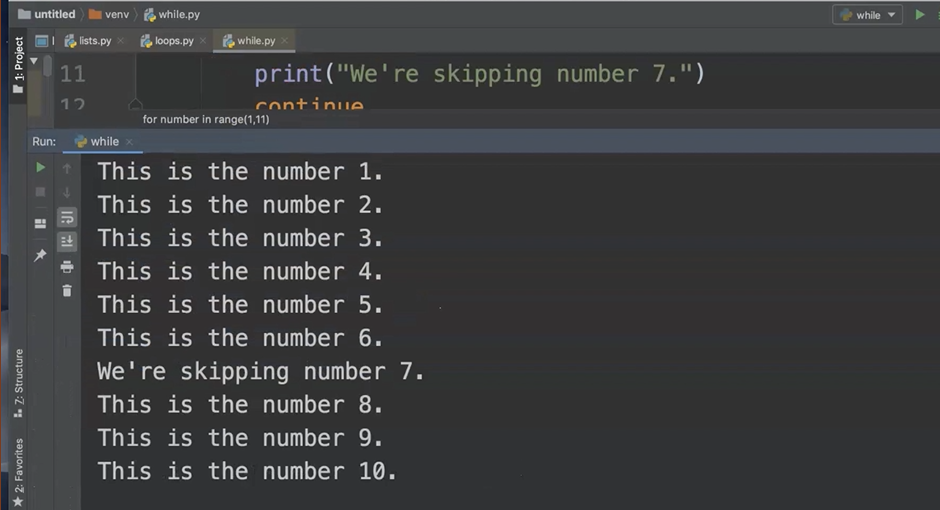You can see we've printed the print line for every number except the number 7 which prints the line in our `if` statement, but then the loop continues on.

Where our `break` statement ended the loop completely once the if condition was met, the `continue` statement does whatever is needed when the if condition is met but then continues the rest of the loop.

### # Loop Control: Pass

Finally, we'll work with the `pass` statement. The `pass` statement allows us to skip over entire parts of our code.

We'll use the `for` loop as our example again, implementing the `pass` statement instead of the `continue` statement.

Again, please note the indentation levels of the `pass` and the `print` statements within the `if` and the `else` block.

``````for number in range(1,11):
if number == 3:
pass
else:
print("The number is {}.".format(number))
``````

Let's take a look and see what output the pass statement provides.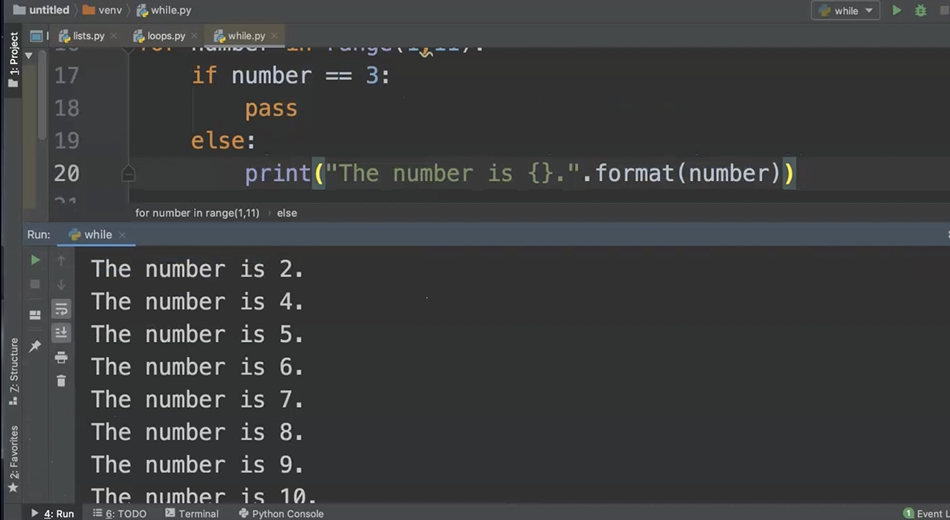You'll notice that the number 3 is skipped completely, so there is no print statement for the number 3.

Every other number in the range is printed with the sentence that we provided.

### # While Loop in Calculator

To work with a `while` loop in a more practical manner, we'll go ahead and work with the calculator functions that we made earlier.

Hopefully you have your file saved and you can return to the code that has your addition, subtraction, multiplication, division functions, as well as the `if`, `elif`, or `else` statements (or you can go to the Git Repo).

In order to make a `while` loop, we're going to create our own “on button”.

We can call this variable “on” and we're going to use `True` with a capital T, which is a Python keyword that delineates Boolean True, and the opposite of that is Boolean False.

Now that we've created our `on` variable and set that variable to `True`, we can go ahead and add our `while` loop.

The one thing you may have noticed when we ran our calculator function the first time is that it ran once and then it ended.

That's not particularly helpful because we want to make sure that like a real calculator, our users can go back and make changes or use the calculator repeatedly if they need to.

So, in order to facilitate this, we'll add a `while` loop here and we can just say `while on`.

Since we've set on equal to `True`, the `on` condition is true. And what this means is that as long as the `on` condition is true, everything that happens inside of our loop will keep happening until on changes to `False`.

In order to make sure all of this functionality happens within the `while` loop, we need to do some indentation.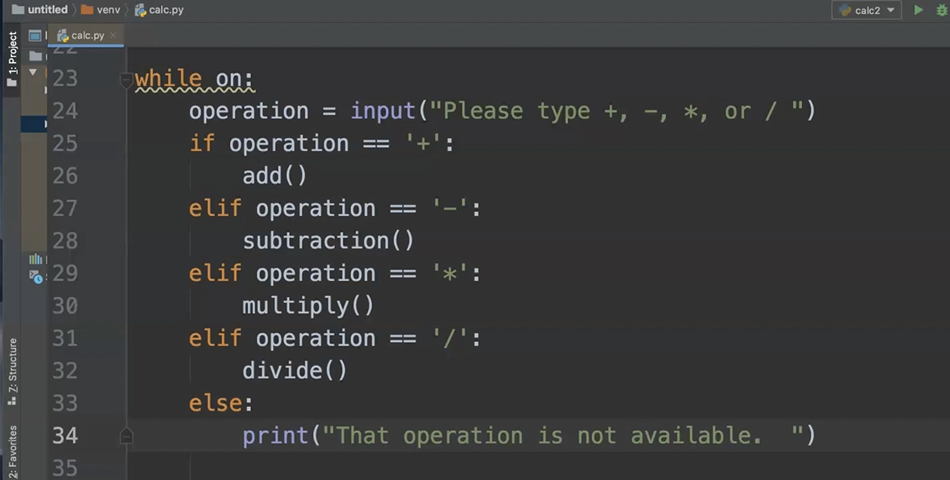NOTE

You'll notice that I've indented everything 4 spaces from where it was originally, so everything that is indented at least 4 spaces is happening inside of the `while` loop and the items that are indented 8 spaces are happening inside of each of the `if`, `elif`, or `else` statements.

In order to provide a quit option, we'll first need to go ahead and add that operation into our options.

I'm just going to go ahead and add “q” as an option that the user can type.

Then I'm going to add an `elif` statement, and in the `elif` statement I am going to set `on` equal to `False`, and I use False with a capital F, so that way that I have my Boolean.

``````on = True
a = float(input("Enter a number. "))
b = float(input("Enter another number. "))
print(a + b)

def subtraction():
a = float(input("Enter a number. "))
b = float(input("Enter another number. "))
print(a - b)

def multiply():
a = float(input("Enter a number. "))
b = float(input("Enter another number. "))
print(a * b)

def divide():
a = float(input("Enter a number. "))
b = float(input("Enter another number. "))
print(a / b)

# enclose selection into while loop

while on:
operation = input("Please type +, -, *, /, or q: ")
if operation == '+':

elif operation == '-':
subtraction()

elif operation == '*':
multiply()

elif operation == '/':
divide()

elif operation == 'q':
calc_on = False

else:
print("That operation is not available.  ")
``````

Let's go ahead and test this out.

First, I'll test out one of the mathematical operations.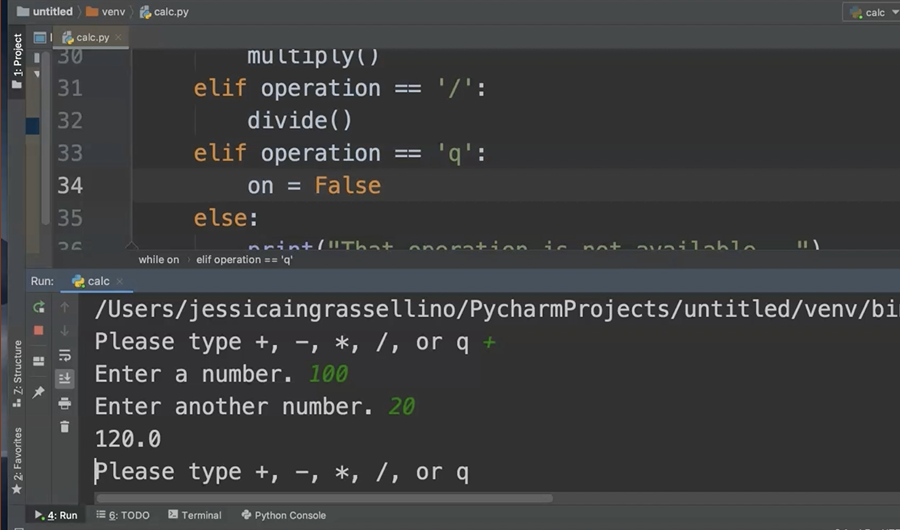I receive the result and the program runs again. So, my `while` loop is working.

Now I want to make sure that random operation is working, and it is.

And now I'd like to quit.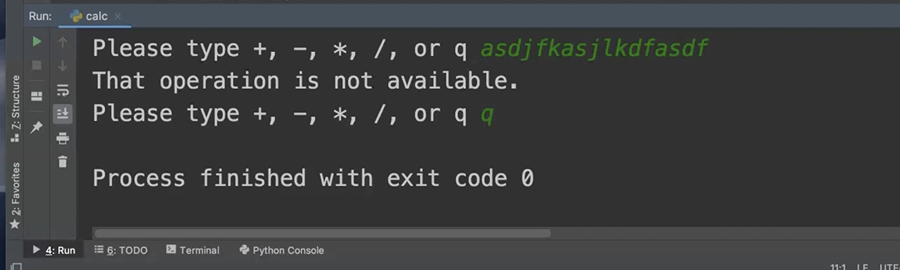With a few easy adjustments and placing the program logic into a `while` loop, we can make our calculator something that has more extensibility and flexibility for the users.